### 通用雪佛兰 赛欧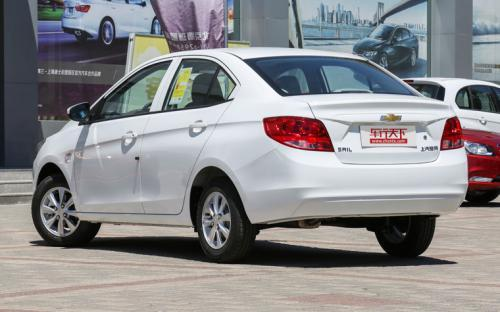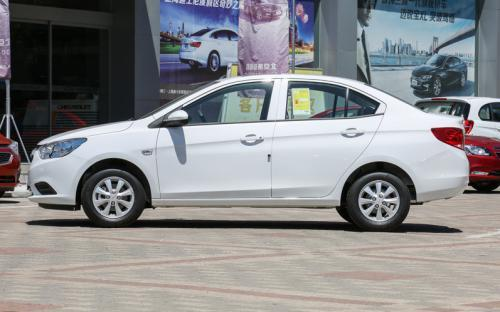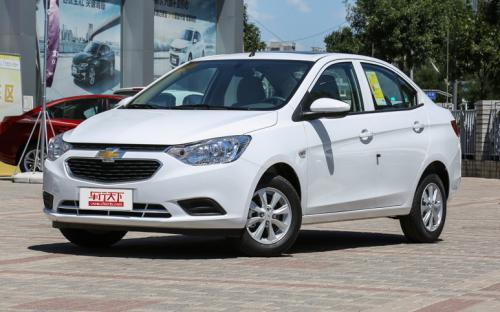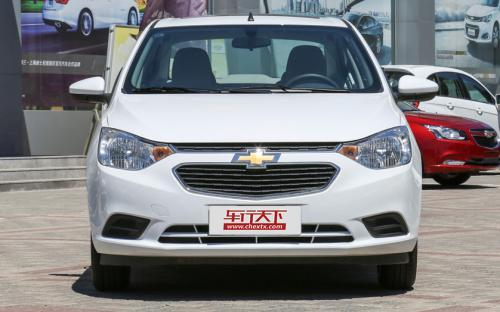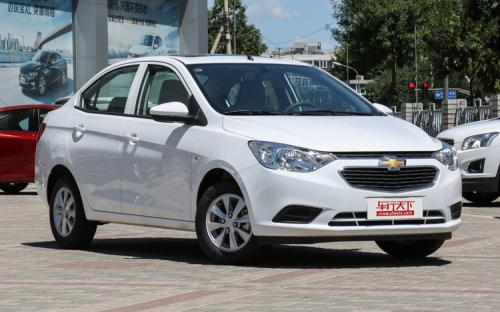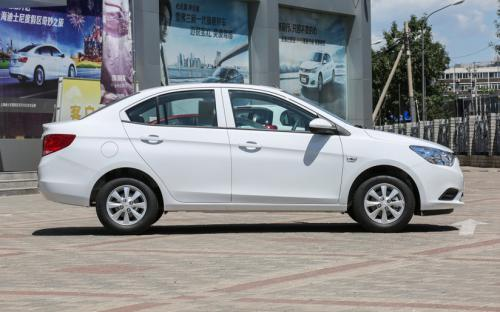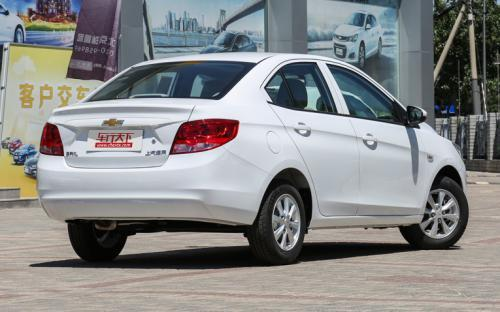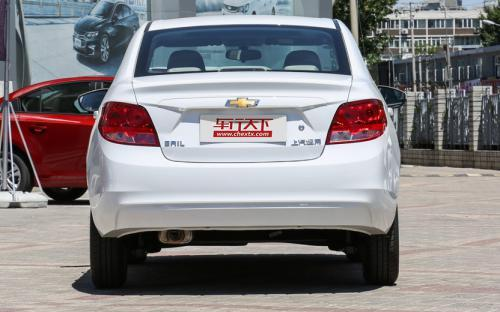0 种颜色可选2016款最低售价：6.39 万元起

4300(mm)1735(mm)1504(mm)##### 配置亮点：
• 胎压监测装置

• ISOFIX儿童座椅接口

• 车身稳定控制(ESC/ESP/DSC等)

• 电动天窗

• 定速巡航

• 后倒车雷达

• 真皮座椅

• GPS导航系统

• 氙气大灯

• 后视镜加热

• 提交
2016款 1.3L 手动舒适天窗版 (82张)

通用雪佛兰 赛欧 绕车实拍• 通用雪佛兰 赛欧 在售车型

排量 车型 厂商指导价 本地最低报价 购车工具
1.3L
6.39万
6.39万

通用雪佛兰 赛欧 经销商

查看更多 >>

### 通用雪佛兰 赛欧 动力加速

赛欧 0-100公里加速时间分布在 12.4-12.4秒 属于 民用级

动力级别 加速时间 车型
民用级(1款)
12.4s
1.3L 手动舒适天窗版

通用雪佛兰 赛欧 视频

通用雪佛兰 赛欧 新闻资讯

# 赛欧3手动舒适天窗版上市 售价6.39万元

上市新车 超过9098次关注

近日，上汽通用宣布，雪佛兰赛欧3正式推出手动舒适天窗版车型，官方指导价6.39万元。新车在手动理想版的基础上增加了天窗及发动机自动启/停功能。

# 赛欧3 AMT自动挡车型上市 享节能惠民补贴

国产新车 超过7791次关注

上海通用汽车宣布雪佛兰赛欧3AMT自动挡车型正式上市，新车提供有3款车型选择，售价区间为6.89-7.99万元。此外，该车还可以享受3000元国家节能惠民补贴。赛欧3AMT车...

# E系列VS赛欧3谁更值得购买

新闻中心 超过4555次关注

随着中国汽车市场的不断发展，各自主品牌也逐渐从消费者心中“低质低价”的形象中走出来，形成了一系列具有竞争力的产品。其中就拿北汽E系列为例，自上市后，不仅累...

# 共4款车型可选 赛欧3将于12月18日上市

国产新车 超过4406次关注

近日我们从上海通用汽车获悉，雪佛兰赛欧3将于12月18日正式上市，新车将提供1.3L和1.5L两种动力共4款车型选择，同时官方还透露了一部分参数配置信息。

# 雪佛兰新赛欧

国产新车 超过3554次关注

我们此前从官方了解，雪佛兰新赛欧将会在广州车展首发。而日前，据国内媒体最新报道，该车有望在12月18日正式上市。

# 试驾上海通用雪佛兰新赛欧 生活新体验

评测 超过3687次关注

赛欧压根就不是一款甘于平庸的车，它的每一次新车亮相，都会引来掌声一片。从2003年的两厢赛欧SRV，开创了中国的平民旅行车概念；到今天的全新赛欧，再一次打破了国...

# 热销家用车型推荐 雪佛兰赛欧三厢版

导购 超过3470次关注

说起赛欧，首先就要说的就是它的价位，作为最便宜的美系合资车，目前在售的国产赛欧已经是第四代改款了，它以亲民的价格、大方的外观和简单的操作赢得了很多消费者...

# 四星车型：雪佛兰新赛欧得41.0分

评测 超过2887次关注

正面100%重叠刚性壁障碰撞试验得分：13.02分，其中头部5分，颈部2分，胸部2.8分，大腿2分，小腿1.22分。

# 雪佛兰-赛欧将换装1.5L发动机 年内上市

新闻 超过2639次关注

现款雪佛兰赛欧自2010年上市至今已有3年时间，而作为合资品牌小型车的标杆车型，其上市后一直有着优秀的市场表现。在同为通用旗下产品的全新别克凯越换装1.5L发动机...

# 通用在印度发布2013款赛欧 外形略作修改

新闻 超过2853次关注

近日，通用汽车在印度发布了2013款赛欧。新车型在外形和内部都有修改，预计将会在不久后上市。

# 新赛欧幸福版上市 售价7.23万-7.93万元

新闻 超过2410次关注

“全民理想家轿”雪佛兰赛欧上周再发四款“幸福版”车型。1.4升手动幸福版与1.4升EMT幸福版的售价分别为7.23万元与7.93万元，两厢、三厢车型同价。北京的雪佛兰经销...

猜你喜欢

﻿
• 快速找车
• 选择品牌
• 选择品牌
• A  奥迪
• A  阿斯顿·马丁
• A  阿尔法·罗密欧
• B  宝沃
• B  布加迪
• B  巴博斯
• B  保时捷
• B  宾利
• B  奔驰
• B  宝马
• B  本田
• B  别克
• B  标致
• B  比亚迪
• B  宝骏
• B  北汽制造
• B  北汽新能源
• B  北汽幻速
• B  北汽威旺
• B  北京汽车
• B  奔腾
• B  北汽绅宝
• C  长安
• C  长安商用
• C  长城
• C  昌河
• D  大众
• D  道奇
• D  DS
• D  东南
• D  东风风神
• D  东风风行
• D  东风小康
• D  东风风度
• D  东风
• F  福特
• F  丰田
• F  菲亚特
• F  法拉利
• F  福田
• F  福迪
• F  福汽启腾
• G  观致
• G  广汽传祺
• G  广汽吉奥
• G  GMC
• H  红旗
• H  汉腾汽车
• H  哈弗
• H  哈飞
• H  海格
• H  海马
• H  华颂
• H  黄海
• H  华泰
• H  恒天
• J  吉利汽车
• J  捷豹
• J  Jeep
• J  江淮
• J  江铃
• J  金杯
• J  九龙
• J  金旅
• K  凯翼
• K  凯迪拉克
• K  克莱斯勒
• K  科尼塞克
• K  卡威
• K  开瑞
• L  路虎
• L  林肯
• L  劳斯莱斯
• L  兰博基尼
• L  雷克萨斯
• L  铃木
• L  雷诺
• L  理念
• L  力帆
• L  莲花汽车
• L  猎豹
• L  路特斯
• L  陆风
• M  马自达
• M  MG
• M  MINI
• M  玛莎拉蒂
• M  摩根
• M  迈凯轮
• N  纳智捷
• O  欧宝
• O  讴歌
• O  欧朗
• Q  奇瑞
• Q  起亚
• Q  启辰
• R  日产
• R  荣威
• R  瑞麒
• S  三菱
• S  斯威汽车
• S  萨博
• S  smart
• S  斯柯达
• S  斯巴鲁
• S  思铭
• S  双龙
• S  上汽大通
• S  双环
• T  特斯拉
• T  腾势
• W  沃尔沃
• W  五菱汽车
• W  五十铃
• W  威兹曼
• W  威麟
• X  现代
• X  雪佛兰
• X  雪铁龙
• X  西雅特
• Y  一汽
• Y  英菲尼迪
• Y  英致
• Y  依维柯
• Y  野马汽车
• Y  永源
• Z  众泰
• Z  中华
• Z  中兴
• Z  知豆
• 选择车系
• 选择车系
• 车型对比
• 选择品牌
• 选择品牌
• A  奥迪
• A  阿斯顿·马丁
• A  阿尔法·罗密欧
• B  宝沃
• B  布加迪
• B  巴博斯
• B  保时捷
• B  宾利
• B  奔驰
• B  宝马
• B  本田
• B  别克
• B  标致
• B  比亚迪
• B  宝骏
• B  北汽制造
• B  北汽新能源
• B  北汽幻速
• B  北汽威旺
• B  北京汽车
• B  奔腾
• B  北汽绅宝
• C  长安
• C  长安商用
• C  长城
• C  昌河
• D  大众
• D  道奇
• D  DS
• D  东南
• D  东风风神
• D  东风风行
• D  东风小康
• D  东风风度
• D  东风
• F  福特
• F  丰田
• F  菲亚特
• F  法拉利
• F  福田
• F  福迪
• F  福汽启腾
• G  观致
• G  广汽传祺
• G  广汽吉奥
• G  GMC
• H  红旗
• H  汉腾汽车
• H  哈弗
• H  哈飞
• H  海格
• H  海马
• H  华颂
• H  黄海
• H  华泰
• H  恒天
• J  吉利汽车
• J  捷豹
• J  Jeep
• J  江淮
• J  江铃
• J  金杯
• J  九龙
• J  金旅
• K  凯翼
• K  凯迪拉克
• K  克莱斯勒
• K  科尼塞克
• K  卡威
• K  开瑞
• L  路虎
• L  林肯
• L  劳斯莱斯
• L  兰博基尼
• L  雷克萨斯
• L  铃木
• L  雷诺
• L  理念
• L  力帆
• L  莲花汽车
• L  猎豹
• L  路特斯
• L  陆风
• M  马自达
• M  MG
• M  MINI
• M  玛莎拉蒂
• M  摩根
• M  迈凯轮
• N  纳智捷
• O  欧宝
• O  讴歌
• O  欧朗
• Q  奇瑞
• Q  起亚
• Q  启辰
• R  日产
• R  荣威
• R  瑞麒
• S  三菱
• S  斯威汽车
• S  萨博
• S  smart
• S  斯柯达
• S  斯巴鲁
• S  思铭
• S  双龙
• S  上汽大通
• S  双环
• T  特斯拉
• T  腾势
• W  沃尔沃
• W  五菱汽车
• W  五十铃
• W  威兹曼
• W  威麟
• X  现代
• X  雪佛兰
• X  雪铁龙
• X  西雅特
• Y  一汽
• Y  英菲尼迪
• Y  英致
• Y  依维柯
• Y  野马汽车
• Y  永源
• Z  众泰
• Z  中华
• Z  中兴
• Z  知豆
• 选择车系
• 选择车系
• 选择车型
• 选择车型
• 意见反馈